# OliNo

Renewable Energy

## Line Lite International BV 4 W 60mm Pear Shape Sharp Ecoled Bulb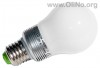presents a light bulb that looks like a normal incandescent (pear shape) light bulb. It needs 230 V AC power, gives as warm white light and consumes about 4 W. This article shows the measurement results. Many parameters are also found in the Eulumdat file.

See this overview for a comparison with other light bulbs.

### Summary measurement data

parameter meas. result remark
Color temperature 2766 K Cold white
Luminous intensity Iv 11 Cd
Beam angle 324 deg
Power P 4.0 W
Power Factor 0.50 For every 1 kWh net power consumed, there has been 1.7 kVAhr for reactive power..
Luminous flux 136 lm
Luminous efficacy 34 lm/W
CRI_Ra 71 Color Rendering Index.
Coordinates chromaticity diagram x=0.4606 and y=0.4197
Fitting E27 This is grid voltage.
D x H external dimensions 59 x 112 mm External dimensions of the pear shaped light bulb.
D x H luminous area 59 x 63 mm Dimensions of the luminous area (used in Eulumdat file). It is the diameter of this matte glass and its height.
General remarks The ambient temperature during the whole set of measurements was 26-26.5 deg C.

Warm up effect: during the warm up time the illuminance and consumed power decreases with 9 %.

Voltage dependency: the power consumption and illumination are not dependent on the voltage.

Measurement report (PDF)Eulumdat fileRight click on icon and save the file.

### Eulumdat light diagram

An interesting graph is the light diagram, indicating the intensity in the C0-C180 and the C90-C270 plane. This light diagram below comes from the program Qlumedit, that extracts these diagrams from an Eulumdat file.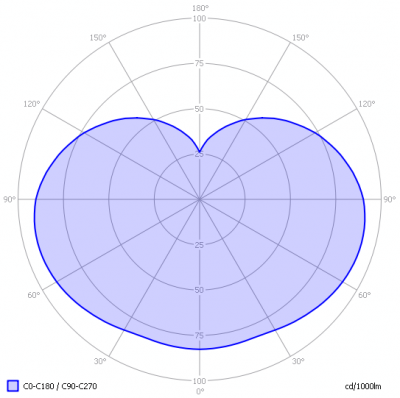The light diagram giving the radiation pattern.

It indicates the luminous intensity around the light bulb. This light bulb has no difference in light diagram between the C0-C180 and C90-C270 plane as the light bulb is symmetrical over its lenth axis (the so called z-axis). C0-C180 and C90-C270 planes are cross sections through the length direction of the light bulb.

The bundle is wide, which is to be expected from a pear shaped light bulb; it emits light all around.

The unit is Cd/1000lm, meaning the intensity in Cd assuming there would be 1000 lumen in the measured light bulb. This enables comparing different types of light bulbs.

### Illuminance Ev at 1 m distance, or luminous intensity Iv

Herewith the plot of the averaged luminous intensity Iv as a function of the inclination angle with the light bulb.The radiation pattern of the light bulb.

This radiation pattern is the average of the light output of the light diagram given earlier. Also, in this graph the luminous intensity is given in Cd.

These averaged values are used (later) to compute the lumen output.Intensity data of every measured turn angle at each inclination angle.

This plot shows per inclination angle the intensity measurement results for each turn angle at that inclination angle. There are little differences in illuminance values for different turn angles. However for further calculations the averaged values will be used. At 180 degrees there is still 30 % of the light output that is present at 0 degree inclination angle.

When using the average values per inclination angle, the beam angle can be computed, being 324 degrees. This is the average value which is valid for any cross section through the z-axis of the light bulb.

### Luminous flux

With the averaged illuminance data at 1 m distance, taken from the graph showing the averaged radiation pattern, it is possible to compute the luminous flux.

The result of this computation for this light spot is a luminous flux of 136 lm.

### Luminous efficacy

The luminous flux being 136, and the power of the lightbulb being 4.0 W, yields a luminous efficacy of 34 lm/W.

A power factor of 0.50 means that for every 1 kWh net power consumed, a reactive component of 1.7 kVAr was needed.

 Light bulb voltage 230 VAC Light bulb current 35 mA Power P 4.0 W Apparent power S 8.0 VA Power factor 0.50

Of this light bulb the voltage across ad the resulting current through it are measured and graphed.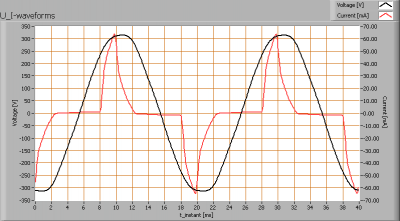Voltage across and current through the lightbulb
There are peaks in current near the tops of the voltage. This results in a waveform for the current that is different from the sineform of the voltage, resulting an a power factor less than 1.
Also the power spectrum of the current is determined.Current power spectrum in % of the first harmonic (50 Hz).

The current peaks result in a current power spectrum with a considerable amount of higher harmonics.

### Color temperature and Spectral power distribution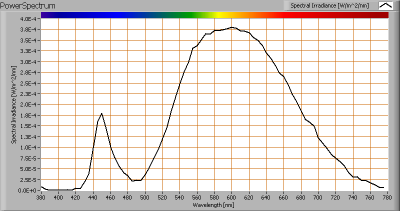The spectral power distribution of this light bulb.

The measured color temperature is about 2750 K which is warm white.

This color temperature is measured straight underneath the light bulb. Below a graph showing the color temperature for different inclination angles.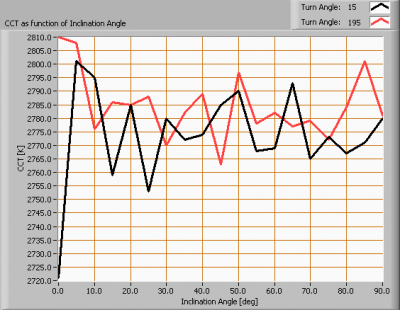Color temperature as a function of inclination angle.

The color temperature is given for inclination angles up to 90 degrees, more is not measured.

The value remains constant within 1 %, which is very constant.

### Chromaticity diagram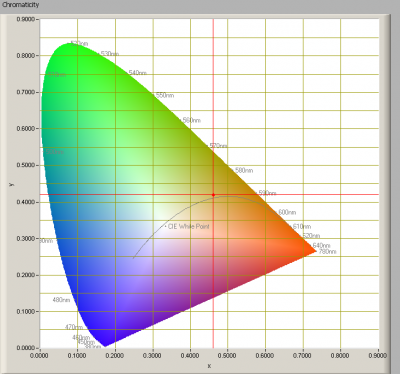The chromaticity space and the position of the lamp’s color coordinates in it.

The light coming from this lamp is at a distance from the Planckian Locus (the black path in the graph).

Its coordinates are x=0.4606 and y=0.4197.

### Color Rendering Index (CRI) or also Ra

Herewith the image showing the CRI as well as how well different colors are represented (rendered). The higher the number, the better the resemblance with the color when a black body radiator would have been used (the sun, or an incandescent lamp).

Each color has an index Rx, and the first 8 indexes (R1 .. R8) are averaged to compute the Ra which is equivalent to the CRI.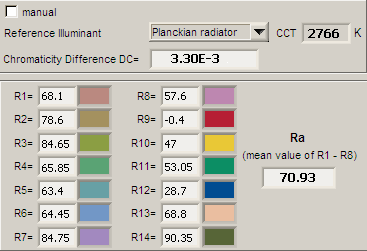CRI of the light of this lightbulb.

The value of 71 is lower than 80 which is considered a minimum value for indoor usage.

Note: the chromaticity difference is 0.0033 indicates the distance to the Planckian Locus. Its value is higher than 0.0054, which means that the calculated CRI result is at the edge of being meaningful.

### Voltage dependency

The dependency of a number of lamp parameters on the lamp voltage is determined. For this, the lamp voltage has been varied and its effect on the following lamp parameters measured: illuminance E_v [lx], the lamppower P [W], the (Correlated) Color Temperature [K] and the luminous efficacy [lm/W].Lamp voltage dependencies of certain light bulb parameters, where the value at 230 V is taken as 100 %.

There is no significant variation of parameters due to the variation of the light bulb voltage, when the voltage varies between 200-250 V.

When the voltage at 230 V varies with + and – 5 V, then the illuminance varies within 0.2 %, so when abrupt voltage changes occur this effect is not visible in the illuminance output.

### Warm up effects

After switch on of a cold lamp, the effect of heating up of the lamp is measured on illuminance E_v [lx], color temperature CT or correlated color temperature CCT [K], the lamppower P [W] and the luminous efficacy [lm/W].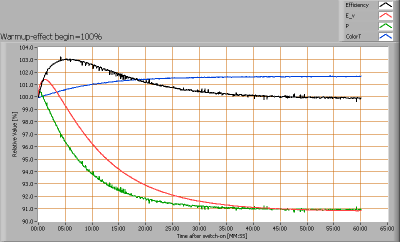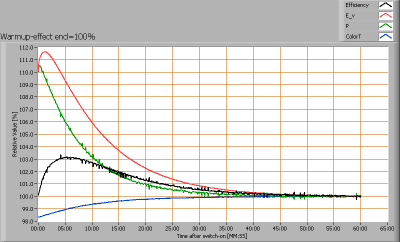Effect of warming up on different light bulb parameters. At top the 100 % level is put at begin, and at bottom at the end.

The warm-up time is about 25 minutes during which the consumed power as well as the illumination level decrease with 9 %